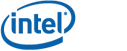# Connectivity Extraction

The connectivity extraction algorithm constructs the connectivity graph where input polygon sets are modeled as graph nodes and assigned node ids and overlap/touching between input polygon sets is modeled as graph edges.  One supported graph formats is std::vector<std::set<int> > where node ids index into the vector and the sets of integers at each index are the ids of nodes for which an edge exists in the graph.  It is required that such vector pre-allocate sufficient elements to store the graph generated by the algorithm, because only the operator[] is used internally to access the graph   The other supported graph format is std::map<int, std::set<int> > which is slightly easier to work with, but potentially more expensive.  Improving the interface to support more generic graph concepts is deferred to future work.

The following is the declaration of the connectivity extraction algorithm.

template <typename coordinate_type>
class connectivity_extraction;

Example code connectivity_extraction_usage.cpp demonstrates using the connectivity extraction algorithm to build a connectivity graph on geometry.

## Member Functions

 connectivity_extraction() Default constructor. connectivity_extraction(      const connectivity_extraction& that) Copy construct. unsigned int insert(const polygon_set_data& ps) Insert a polygon set graph node, the value returned is the id of the graph node. template unsigned int insert(const GeoObjT& geoObj) Insert a geometry object that is a refinement of polygon set as a graph node, the return value is the id of the graph node. template void extract(GraphT& graph) Accepts a graph object that conforms to the expectations defined above.  Performs connectivity extraction and populates the graph object.

Copyright: Copyright © Intel Corporation 2008-2010. Distributed under the Boost Software License, Version 1.0. (See accompanying file LICENSE_1_0.txt or copy at http://www.boost.org/LICENSE_1_0.txt)